﻿ Python Projects: Compute the value of Pi to n number of decimal places - w3resource# Python Projects: Compute the value of Pi to n number of decimal places

## Python Project-1 with Solution

Create a Python project to get the value of Pi to n number of decimal places.
Note: Input a number and the program will generate PI to the nth digit

Sample Solution -1 :

Python Code:

``````#!/usr/bin/env python3
# https://github.com/MrBlaise/learnpython/blob/master/Numbers/pi.py
# Find PI to the Nth Digit
# Have the user enter a number 'n'
# and print out PI to the 'n'th digit

def calcPi(limit):  # Generator function
"""
Prints out the digits of PI
until it reaches the given limit
"""

q, r, t, k, n, l = 1, 0, 1, 1, 3, 3

decimal = limit
counter = 0

while counter != decimal + 1:
if 4 * q + r - t < n * t:
# yield digit
yield n
# insert period after first digit
if counter == 0:
yield '.'
# end
if decimal == counter:
print('')
break
counter += 1
nr = 10 * (r - n * t)
n = ((10 * (3 * q + r)) // t) - 10 * n
q *= 10
r = nr
else:
nr = (2 * q + r) * l
nn = (q * (7 * k) + 2 + (r * l)) // (t * l)
q *= k
t *= l
l += 2
k += 1
n = nn
r = nr

def main():  # Wrapper function

# Calls CalcPi with the given limit
pi_digits = calcPi(int(input(
"Enter the number of decimals to calculate to: ")))

i = 0

# Prints the output of calcPi generator function
# Inserts a newline after every 40th number
for d in pi_digits:
print(d, end='')
i += 1
if i == 40:
print("")
i = 0

if __name__ == '__main__':
main()
``````

Sample Output:

```Enter the number of decimals to calculate to: 5
3.14159
```

Flowchart: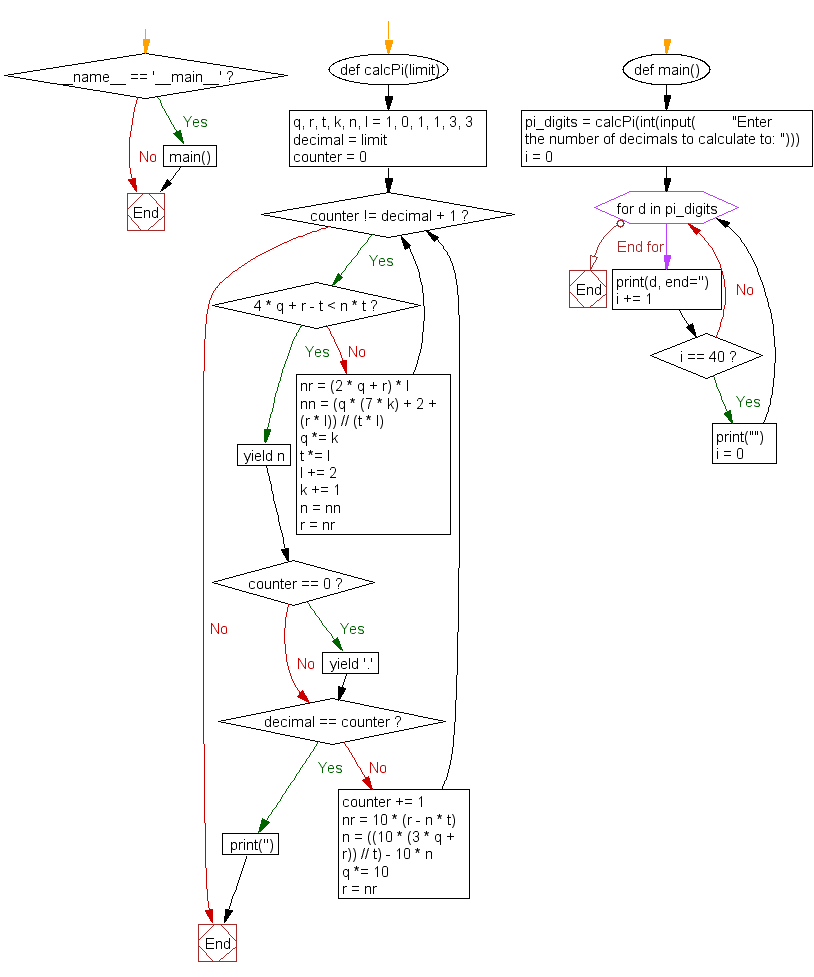Sample Solution -2 :

Python Code:

``````#https://github.com/rlingineni/PythonPractice/blob/master/piCalc/pi.py
import math

def CalculatePi(roundVal):

somepi = round(math.pi,roundVal);
pi = str(somepi)
someList = list(pi)
return somepi;
roundTo = input('Enter the number of digits you want after the decimal for Pi: ')
try:
roundint = int(roundTo);
print(CalculatePi(roundint));
except:
print("You did not enter an integer");
``````

Sample Output:

```Enter the number of digits you want after the decimal for Pi: 5
3.14159
```

Flowchart: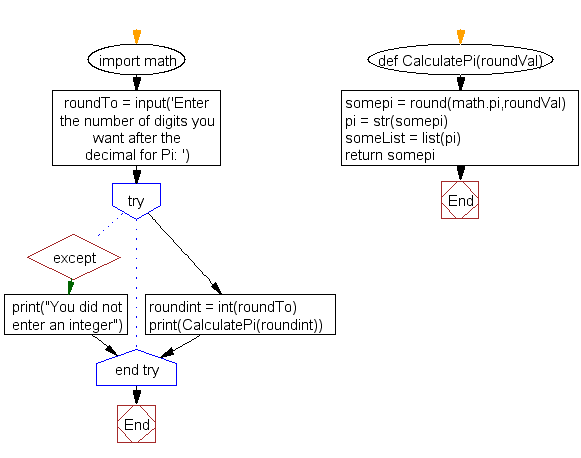Sample Solution -3 :

Python Code:

``````# generate pi to nth digit
# Chudnovsky algorihtm to find pi to n-th digit
# from https://en.wikipedia.org/wiki/Chudnovsky_algorithm
# https://github.com/microice333/Python-projects/blob/master/n_digit_pi.py

import decimal
def compute_pi(n):
decimal.getcontext().prec = n + 1
C = 426880 * decimal.Decimal(10005).sqrt()
K = 6.
M = 1.
X = 1
L = 13591409
S = L
for i in range(1, n):
M = M * (K ** 3 - 16 * K) / ((i + 1) ** 3)
L += 545140134
X *= -262537412640768000
S += decimal.Decimal(M * L) / X
pi = C / S
return pi

while True:
n = int(input("Please type number between 0-1000: "))
if n >= 0 and n <= 1000:
break
print(compute_pi(n))
``````

Sample Output:

```Please type number between 0-1000: 8
3.14159266
```

Flowchart: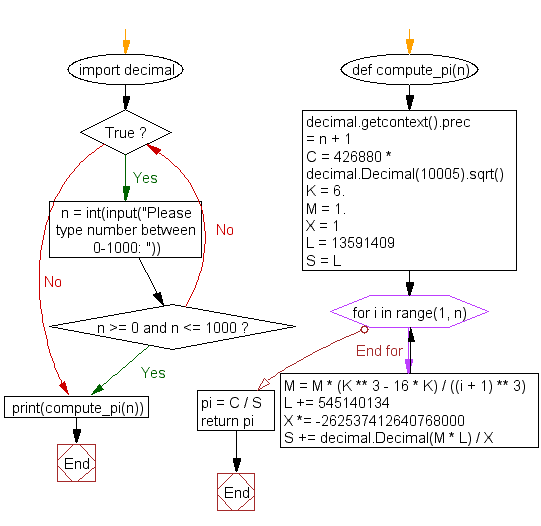Sample Solution -4 :

Python Code:

``````#coding:utf-8
"""
Pi = SUM k=0 to infinity 16^-k [ 4/(8k+1) - 2/(8k+4) - 1/(8k+5) - 1/(8k+6) ]
ref: https://www.math.hmc.edu/funfacts/ffiles/20010.5.shtml
https://github.com/Flowerowl/Projects/blob/master/solutions/numbers/find_pi_to_the_nth_digit.py
"""
from __future__ import division
import math
from decimal import Decimal as D
from decimal import getcontext

getcontext().prec = 400
MAX = 10000
pi = D(0)

for k in range(MAX):
pi += D(math.pow(16, -k)) * (D(4/(8*k+1)) - D(2/(8*k+4)) - D(1/(8*k+5)) - D(1/(8*k+6)))

print('PI >>>>>>>>>>' , pi)
```
```

Sample Output:

```PI >>>>>>>>>> 3.141592653589793235602541812141379285866306715610149218684551786650252153469632637309117852151159714537117427945211584672226312439842050377685609241701572487956515336256451884653308025999156699529458184376955252001085693453737649800187605123943826169924919030970189770760788301321900624395693702676234864995384812854310220642057305637554086524948960077795499941378034295271204385281577283147587536626
```

Flowchart: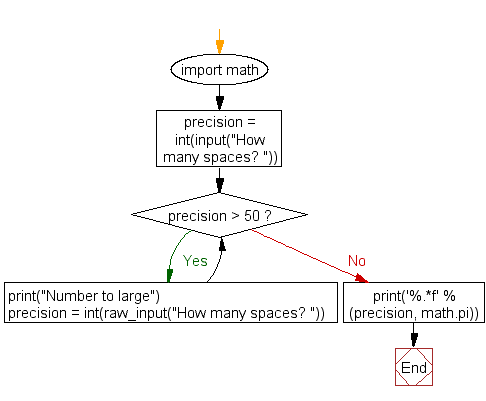Sample Solution -5 :

Python Code:

``````""""Find PI to the Nth Digit -
https://bitbucket.org/desertwebdesigns/learn_python/src/master/Numbers/pi.py?fileviewer=file-view-default

Enter a number and have the program generate PI up to that many decimal places.
Keep a limit to how far the program will go."""

import math

precision = int(input("How many spaces? "))

while precision > 50:
print("Number to large")
precision = int(raw_input("How many spaces? "))
else:
print('%.*f' % (precision, math.pi))
``````

Sample Output:

```How many spaces? 5
3.14159
```

Flowchart: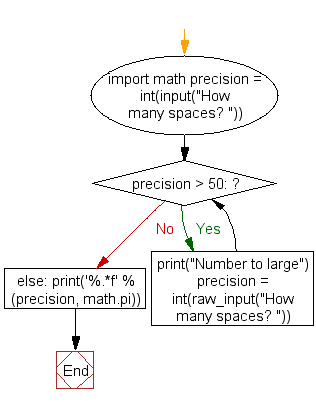Improve this sample solutions and post your code through Disqus

﻿